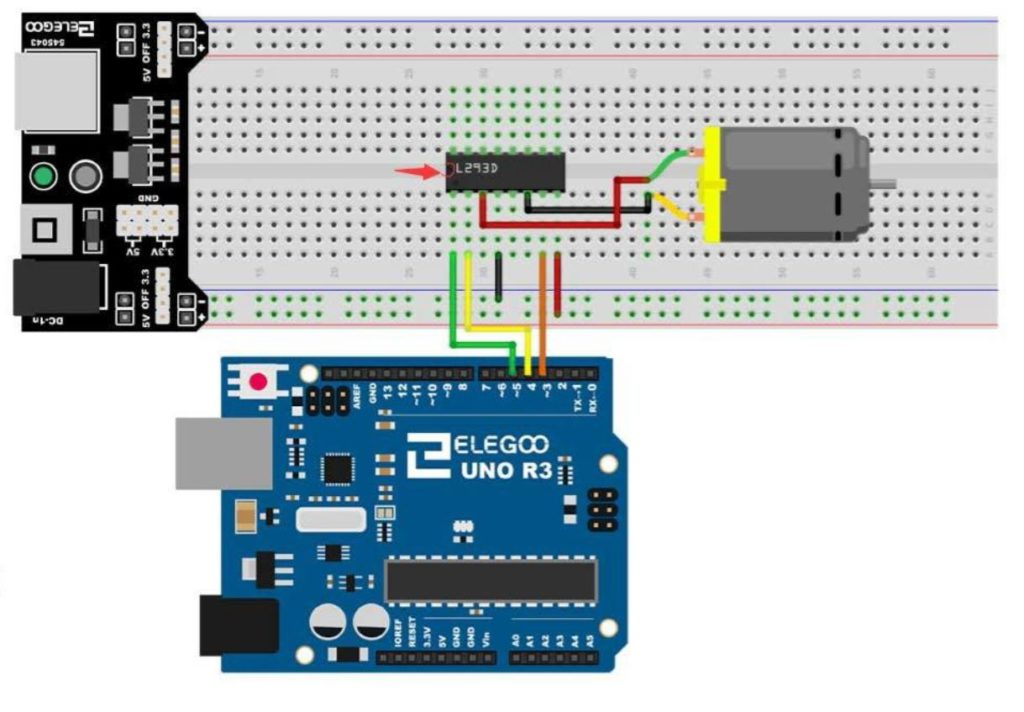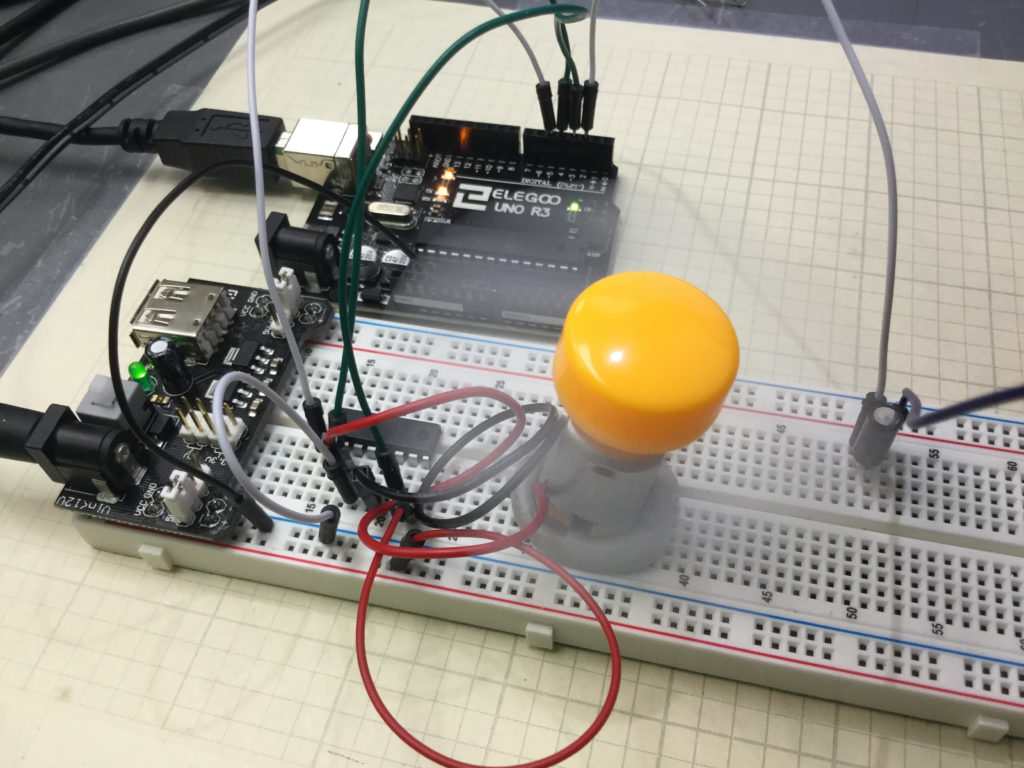# Robotics Training LESSON 5: Programming Core DC Motor Control Functions

In this video tutorial, we show how to program the Elegoo Smart Car 3 to have simple core capabilities. We create code that will move the car forward or backward by a user defined distance. We also have functions that allow the car to turn by a user defined angle. With these simple functions, we can program the car to move along a path we define. Below is the code we developed in this video.

# ROBOTICS Training LESSON 4: Fundamentals of Robot Motor Control Using L298N Module

In the video tutorial above we describe the fundamentals of Robot Motor Control Using the L298N control module. This establishes the core functions that will be used throughout the remainder of these lessons. We show how we can create the core motion components of forward, backward, turn left and turn right. The code developed in the lesson above is presented below for your convenience. If you would like to play along at home, you can get the hardware I am using HERE:

# Arduino Tutorial 39: Using a Joystick to Control DC Motor Speed and DirectionThis is a simple example of how to control speed and direction of a DC motor with a joystick.

Lesson 37 and 38 showed some preliminary concepts in controlling a DC motor using an arduino and the L293D motor controller. In the video above we show how to control the speed and direction of a simple DC motor using a joystick. In the neutral position, the motor is stationary. Then the speed smoothly increases as you move the joystick forward, until you reach maximum speed.

Lesson 37 and 38 showed some preliminary concepts in controlling a DC motor using an arduino and the L293D motor controller. In the video above we show how to control the speed and direction of a simple DC motor using a joystick. In the neutral position, the motor is stationary. Then the speed smoothly increases as you move the joystick forward, until you reach maximum speed. Similarly, in pulling the joystick back from the neutral position, the motor gradually increases speed in the reverse direction. The diagram below shows the basic motor control schematic we are working from.Connection Diagram for a DC Motor Controller using the L293 Control Chip

In the circuit diagram above, we also add a wire to connect the arduino ground to the power supply ground. It is always good to have all components connected to a common ground rail. Of course, you also need to add the joystick controller as shown in the video.

This is the code we used in this

# Arduino Tutorial 38: Using a Tilt Switch Cut Off With a DC MotorDC Motor circuit with a tilt switch cutoff

In this lesson we explore how to add a tilt cutoff to our DC motor project. In many cases, you may want to automatically turn your motors off in the case where your project or robot tips over. The tilt switch is a simple way to do this. The video below takes you through the process step-by-step.

If you need help hooking the circuit up, the diagram below shows you a schematic of what we are working with. All these components are part of our ELEGOO Super Starter Kit.Connection Diagram for a DC Motor Controller using the L293 Control Chip

In addition, you will need to add the tilt switch, as shown in the video.

Below is the code we used, with the tilt switch connected to pin 2 on the arduino.